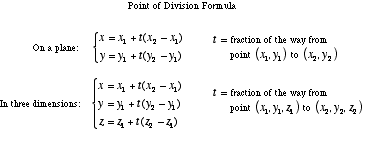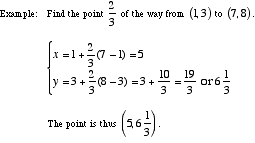# point of division formula

Point of Division Formula

The formula for the coordinates of a point which part of the way from one point to another.

Note: The midpoint formula is a special case of the point of division formula in which t = ½.